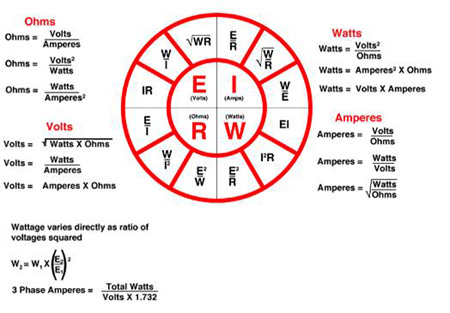# Ohm's Law

The physicist George Ohm described in 1827 measurements of applied voltage and current passing through electrical circuits containing various length of wire.
Ohm's law states that the current (I) passing through a conductor between two points is directly proportional to the voltage (V) and inversely proportional to the resistance (R).
The mathematical equation for this relationship is: I= V / R

It is an extremely useful equation not just in the field of industrial heating applications.
Here are a couple schematics for basic electrical connections:Home Basic Industrial Heating Know How Technical Calculations for Industrial Heating Thermal Energy Costs Standard and Norms Technical Papers Credit Application RFQ and Contact Us

# Ohm's Law

The physicist George Ohm described in 1827 measurements of applied voltage and current passing through electrical circuits containing various length of wire.
Ohm's law states that the current (I) passing through a conductor between two points is directly proportional to the voltage (V) and inversely proportional to the resistance (R).
The mathematical equation for this relationship is: I= V / R

It is an extremely useful equation not just in the field of industrial heating applications.
Here are a couple schematics for basic electrical connections: